Latest Banking jobs   »   Quantitative Aptitude Quiz For SBI PO,...

# Quantitative Aptitude Quiz For SBI PO, Clerk Prelims 2021- 14th June

Directions (1-5): In each question two equations numbered (I) and (II) are given. You should solve both the equations and mark appropriate answer.
(a) If x = y or no relation can be established
(b) If x > y
(c) If x < y
(d) If x ≥ y
(e) If x ≤ y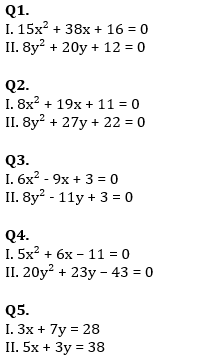Directions (6-10): In each question two equations numbered (I) and (II) are given. Student should solve both the equations and mark appropriate answer.
(a) If x = y or no relation can be established
(b) If x > y
(c) If x < y
(d) If x ≥ y
(e) If x ≤ y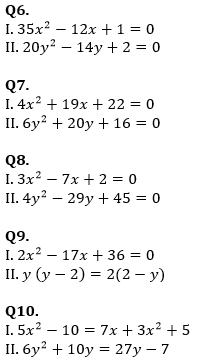Directions (11-15): In each of these questions, two equations (I) and (II) are given. You have to solve both the equations and give answer
(a) if x > y
(b) if x ≥ y
(c) if x < y
(d) if x ≤ y
(e) if x = y or no relation can be established between x and y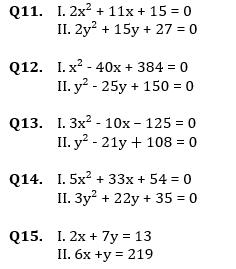Practice More Questions of Quantitative Aptitude for Competitive Exams:

###### SBI Clerk Study Plan 2021

Solutions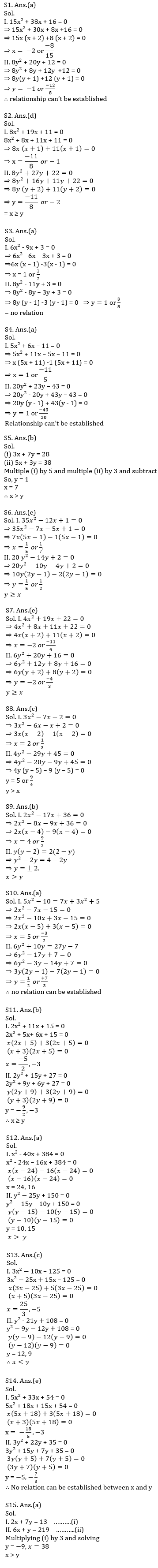#### Congratulations!

Incorrect details? Fill the form again here

•Quantitative Aptitude Quiz For SBI Clerk...
•English Quizzes For SBI Clerk Prelims 20...
•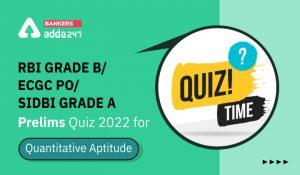Quantitative Aptitude Quiz For RBI Grade...
•Reasoning Ability Quiz For SBI Clerk Pre...
•Quantitative Aptitude Quiz For SBI Clerk...
•Quantitative Aptitude Quiz For SBI Clerk...